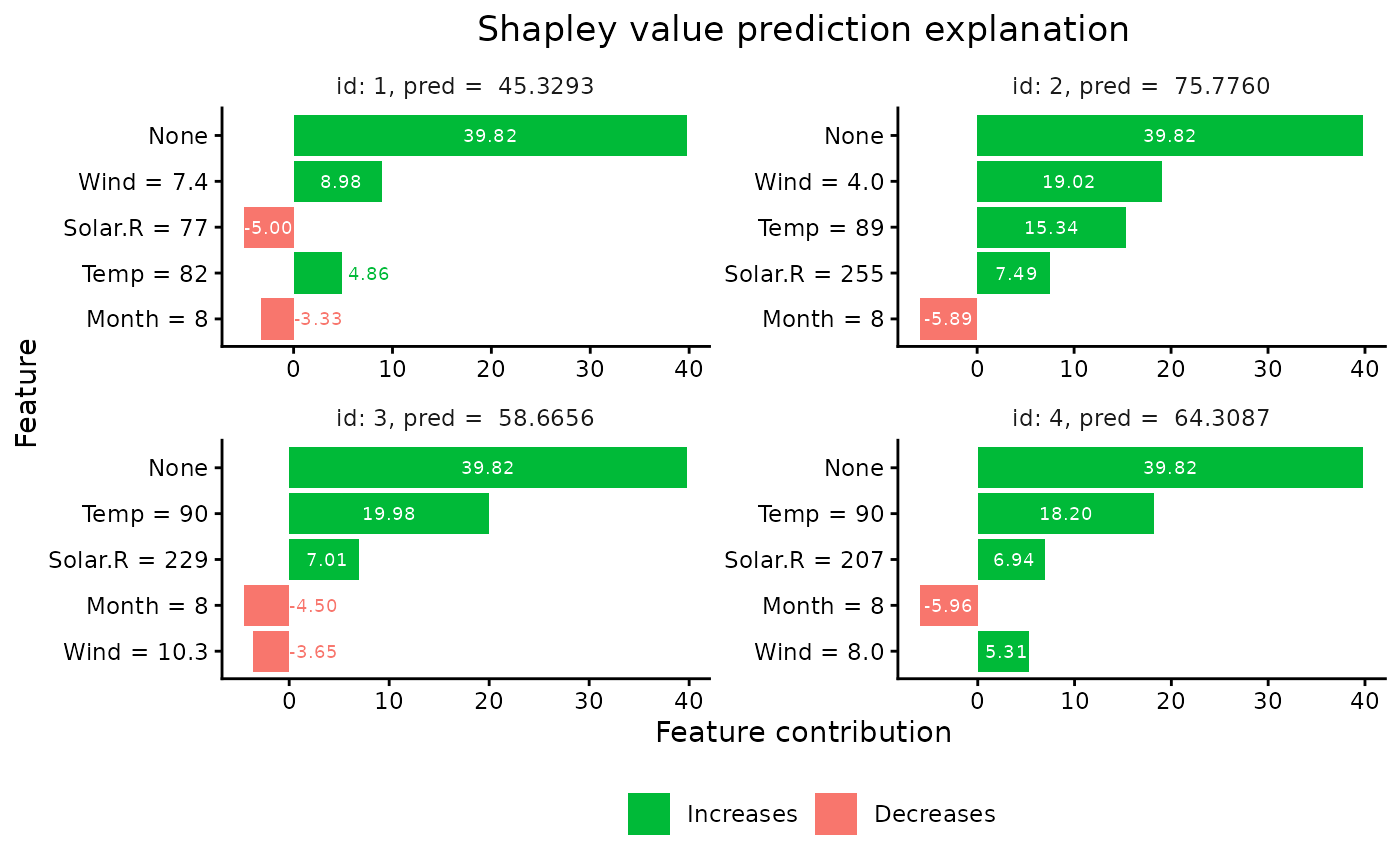Plots the individual prediction explanations.

# S3 method for shapr
plot(
x,
digits = 3,
plot_phi0 = TRUE,
index_x_test = NULL,
top_k_features = NULL,
...
)

## Arguments

x

An shapr object. See explain.

digits

Integer. Number of significant digits to use in the feature description

plot_phi0

Logical. Whether to include phi0 in the plot

index_x_test

Integer vector. Which of the test observations to plot. E.g. if you have explained 10 observations using explain, you can generate a plot for the first 5 observations by setting index_x_test = 1:5.

top_k_features

Integer. How many features to include in the plot. E.g. if you have 15 features in your model you can plot the 5 most important features, for each explanation, by setting top_k_features = 1:5.

...

Currently not used.

## Value

ggplot object with plots of the Shapley value explanations

## Details

See vignette("understanding_shapr", package = "shapr") for an example of how you should use the function.

Martin Jullum

## Examples

if (requireNamespace("MASS", quietly = TRUE)) {
data("Boston", package = "MASS")

# Split data into test- and training data
x_test <- tail(Boston, 3)

# Fit a linear model
model <- lm(medv ~ lstat + rm + dis + indus, data = x_train)

# Create an explainer object
explainer <- shapr(x_train, model)

# Explain predictions
p <- mean(x_train\$medv)

# Empirical approach
explanation <- explain(x_test,
explainer,
approach = "empirical",
prediction_zero = p,
n_samples = 1e2
)

if (requireNamespace("ggplot2", quietly = TRUE)) {
# Plot the explantion (this function)
plot(explanation)
}
}
#>
#> Success with message:
#> The columns(s) crim, zn, chas, nox, age, rad, tax, ptratio, black, medv is not used by the model and thus removed from the data.
#>
#> Success with message:
#> The columns(s) crim, zn, chas, nox, age, rad, tax, ptratio, black, medv is not used by the model and thus removed from the data.